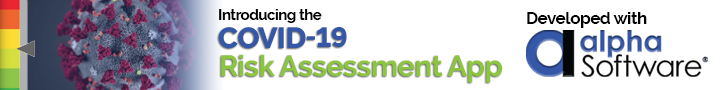# Thread: Checksum Algorithm for Code128

1. ##Checksum Algorithm for Code128

Has anyone ever written an Xbasic script for calculating Modulo 103 checksums on Code128 barcodes? If you have and wouldn't minde sharing the code, I'd be grateful. If you can pint me in the right direction, that would be nice too.

Searching produced no results, and the checksum function in A5 v5/6 doesn't do Mod103.Reply With Quote

2. ##RE: Checksum Algorithm for Code128

I did something way long ago that's in the code archive, search for code 128. If I remember correctly I only implemented it for an even number of characters in the input string as I was working with encoding twelve digit upc numbers.Reply With Quote

3. ##RE: Checksum Algorithm for Code128

And I just adapted this from something I found on the net.

FUNCTION cksm128 AS C (input_string AS C )
'*
'* Initialize running total with value of start character, then scan the
'* string and add character value times position
'*
SUBTOT = 104
FOR I = 1 TO LEN(input_string)
C = SUBSTR(input_string, I, 1)
X = asc(C) - 32
SUBTOT = SUBTOT + (X * I)
NEXT I
'*
'* Calculate Modulo 103. This is the checksum
'*
cksm128= MOD(SUBTOT,103)
'*
'* Now convert numeric checksum to a character. This conversion takes
'* into account the particular mapping of the font being used (this
'*
''select CASE
'' CASE CHKDIG = 0
'' CHKCHR = CHR(174)
'' CASE CHKDIG " 94
'' CHKCHR = CHR(CHKDIG + 32)
'' OTHERWISE
'' CHKCHR = CHR(CHKDIG + 71)
''ENDCASE
''*
''* Now format the final output string: start character,
''* data, check character, and stop character.
''*
''OUTSTRING = CHR(162) + MYSTRING + CHKCHR + CHR(164)
END FUNCTION

I left in some other parts than the checksum "value" which you might be able to use. Usage is

? cksm128("BarCode 1")
= "33"Reply With Quote

4. ##RE: Checksum Algorithm for Code128

The function in the archive is limited to subset C while I think this one handles B and C.Reply With Quote

5. ##RE: Checksum Algorithm for Code128

Stan:

Thanks for your help. This will get me started.Reply With Quote

6. ##Re: Checksum Algorithm for Code128

I notice that the checksum formula states the the Code 128 start character is 104 and the value of 104 is used in calculating the checksum. Why does the routine then use CHR(162) as the start character instead of CHR(104) ?

I am having trouble generating readable code128 barcodes in some cases.

I have also seen this checksum calculation shown as

if 0 then 194
else if < 64 then +32
else +100

if 0 then 174
ekse uf < 64 then +32
else +71

Why are there two different formulas?

Any help would be appreciated.

Thanks.

PaulReply With Quote

7. ##Re: Checksum Algorithm for Code128

'* Now convert numeric checksum to a character. This conversion takes
'* into account the particular mapping of the font being used
(thisReply With Quote

8. ##Re: Checksum Algorithm for Code128

I think this passage concerning generating Code 128 barcodes in .NET may be helpful.Reply With Quote

####Posting Permissions

• You may not post new threads
• You may not post replies
• You may not post attachments
• You may not edit your posts
•